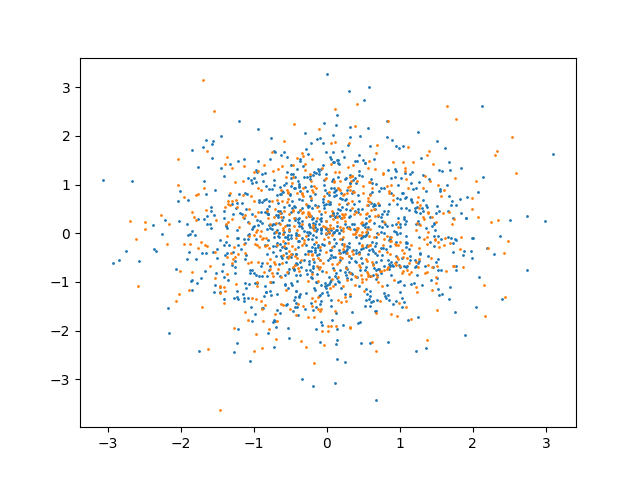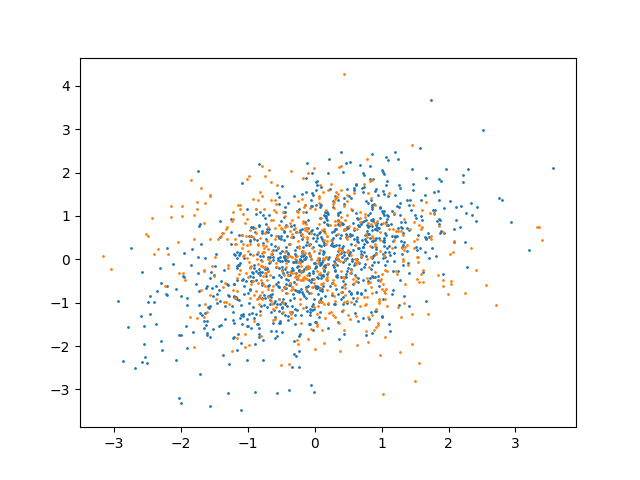# The energy distance test of homogeneity#

Example that shows the usage of the energy distance test.

import matplotlib.pyplot as plt
import numpy as np
import pandas as pd

import dcor


Given samples (of possible different sizes) of several random vectors with the same arbitrary dimension, the energy distance can be used to construct a permutation test of homogeneity. The null hypothesis $$\mathcal{H}_0$$ is that the two random vectors have the same distribution, where the alternative hypothesis $$\mathcal{H}_1$$ is that their distributions differ.

As an example, we can consider a case with identically distributed data:

n_samples_x = 1000
n_samples_y = 600
random_state = np.random.default_rng(63263)

x = random_state.multivariate_normal(np.zeros(2), np.eye(2), size=n_samples_x)
y = random_state.multivariate_normal(np.zeros(2), np.eye(2), size=n_samples_y)

plt.scatter(x[:, 0], x[:, 1], s=1)
plt.scatter(y[:, 0], y[:, 1], s=1)
plt.show()

dcor.homogeneity.energy_test(
x,
y,
num_resamples=200,
random_state=random_state,
)HypothesisTest(pvalue=0.9502487562189055, statistic=0.730988457642523)


Under the null hypothesis, the p-value would have a uniform distribution between 0 and 1. Under the alternative hypothesis, the p-value would tend to 0. Thus, it is common to reject the null hypothesis when the p-value is below a predefined threshold $$\alpha$$ (the significance level). There is thus a probability $$\alpha$$ of rejecting the null hypothesis even when it is true (Type I error). To ensure that this does not happen often one typically chooses a value for $$\alpha$$ of 0.05 or 0.01, to obtain a Type I error less than 5% or 1% of the time, respectively. In this case as the p-value is greater than the threshold we (correctly) don’t reject the null hypothesis, and thus we would consider the random variables independent.

We can now consider the following data:

x = random_state.multivariate_normal(
np.zeros(2),
[
[1, 0.5],
[0.5, 1],
],
size=n_samples_x,
)
y = random_state.multivariate_normal(np.zeros(2), np.eye(2), size=n_samples_y)

plt.scatter(x[:, 0], x[:, 1], s=1)
plt.scatter(y[:, 0], y[:, 1], s=1)
plt.show()Now the two distributions have different variance. Thus, the test should reject the null hypothesis:

dcor.homogeneity.energy_test(
x,
y,
num_resamples=200,
random_state=random_state,
)

HypothesisTest(pvalue=0.004975124378109453, statistic=8.192156215078683)


We can see that the p-value obtained is indeed very small, and thus we can safely reject the null hypothesis, and consider that the distributions are very different.

The test illustrated here is a permutation test, which compares the distance covariance of the original dataset with the one obtained after random permutations of one of the input arrays. Thus, increasing the number of permutations makes the p-value more accurate, but increases the computational cost. With a low number of permutations or low number of observations, it is even possible to not reject the true hypothesis when it is not true (Type II error).

We can now check how this test control effectively the Type I and Type II errors. We can do a simple Monte Carlo test, as explained in the Example 1 of Székely and Rizzo1. What follows is a replication of the results obtained in that example, using a lower number of test repetitions due to time constraints. Users are encouraged to download this example and increase that number to obtain better estimates of the Type I and Type II errors. In order to replicate the original results, one should set the value of n_tests to 10000 and num_resamples to 499.

We generate data from two uncorrelated multivariate normal distributions, with means $$(0, 0)$$ and $$(0, \delta)$$. For $$\delta = 0$$ the two random vectors have the same distribution, and thus we can check the Type I error. In all the other cases we can check the Type II error for a particular value of $$\delta$$.

n_tests = 100
n_obs_list = [10, 15, 20, 25, 30, 40, 50, 75, 100]
num_resamples = 200
significance = 0.1

def multivariate_normal(n_obs, delta):
return random_state.multivariate_normal(
[0, delta],
np.eye(2),
size=n_obs,
)

deltas = [0, 0.5, 0.75, 1]
table = pd.DataFrame()
table["n₁"] = n_obs_list
table["n₂"] = n_obs_list

for delta in deltas:
dist_results = []
for n_obs in n_obs_list:
n_errors = 0
for _ in range(n_tests):
x = multivariate_normal(n_obs, 0)
y = multivariate_normal(n_obs, delta)

test_result = dcor.homogeneity.energy_test(
x,
y,
num_resamples=num_resamples,
random_state=random_state,
)

if test_result.pvalue < significance:
n_errors += 1

error_prob = n_errors / n_tests
dist_results.append(error_prob)

table[f"δ = {delta}"] = dist_results

table

n₁ n₂ δ = 0 δ = 0.5 δ = 0.75 δ = 1
0 10 10 0.11 0.20 0.43 0.47
1 15 15 0.08 0.25 0.53 0.77
2 20 20 0.07 0.36 0.59 0.82
3 25 25 0.13 0.42 0.71 0.92
4 30 30 0.06 0.47 0.79 0.98
5 40 40 0.07 0.59 0.91 0.98
6 50 50 0.09 0.71 0.93 1.00
7 75 75 0.13 0.82 0.99 1.00
8 100 100 0.14 0.95 1.00 1.00

## Bibliography#

1

G. Székely and M. L. Rizzo. Testing for equal distributions in high dimensions. InterStat, 5(16.10):1249–1272, 2004. URL: http://ip.statjournals.net:2002/InterStat/ARTICLES/2004/abstracts/0411005.html-ssi.

Total running time of the script: (1 minutes 21.114 seconds)

Gallery generated by Sphinx-Gallery